CBSE Class 10 Sample Paper for 2020 Boards - Maths Standard

Class 10
Solutions of Sample Papers for Class 10 Boards

## Two friends Seema and Aditya work in the same office at Delhi. In the Christmas vacations, both decided to go to their hometowns represented by Town A and Town B respectively in the figure given below. Town A and Town B are connected by trains from the same station C (in the given figure)in Delhi. Based on the given situation, answer the following questions: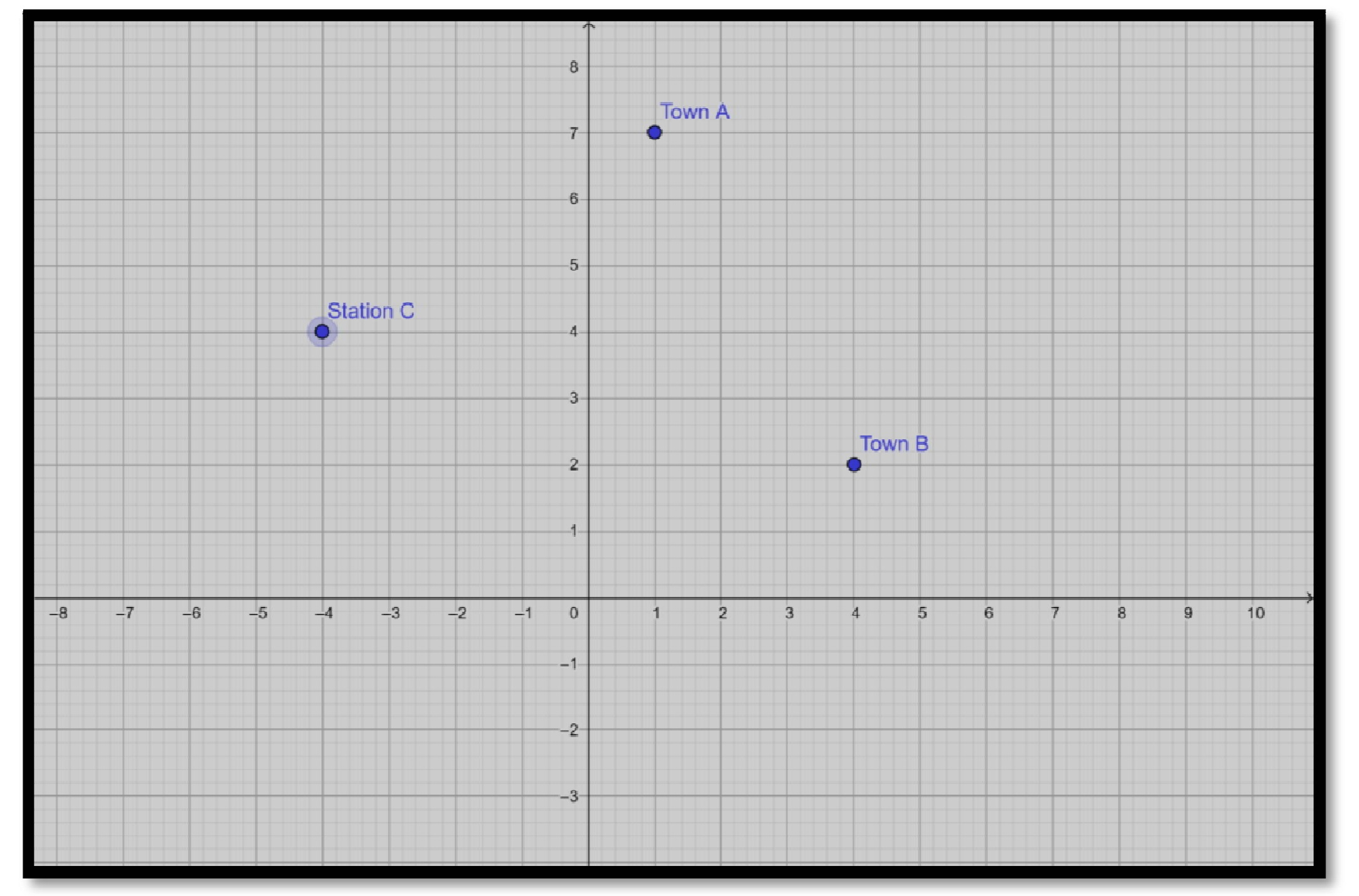## (iii) Find the area of the triangle formed by joining the points represented by A, B and C.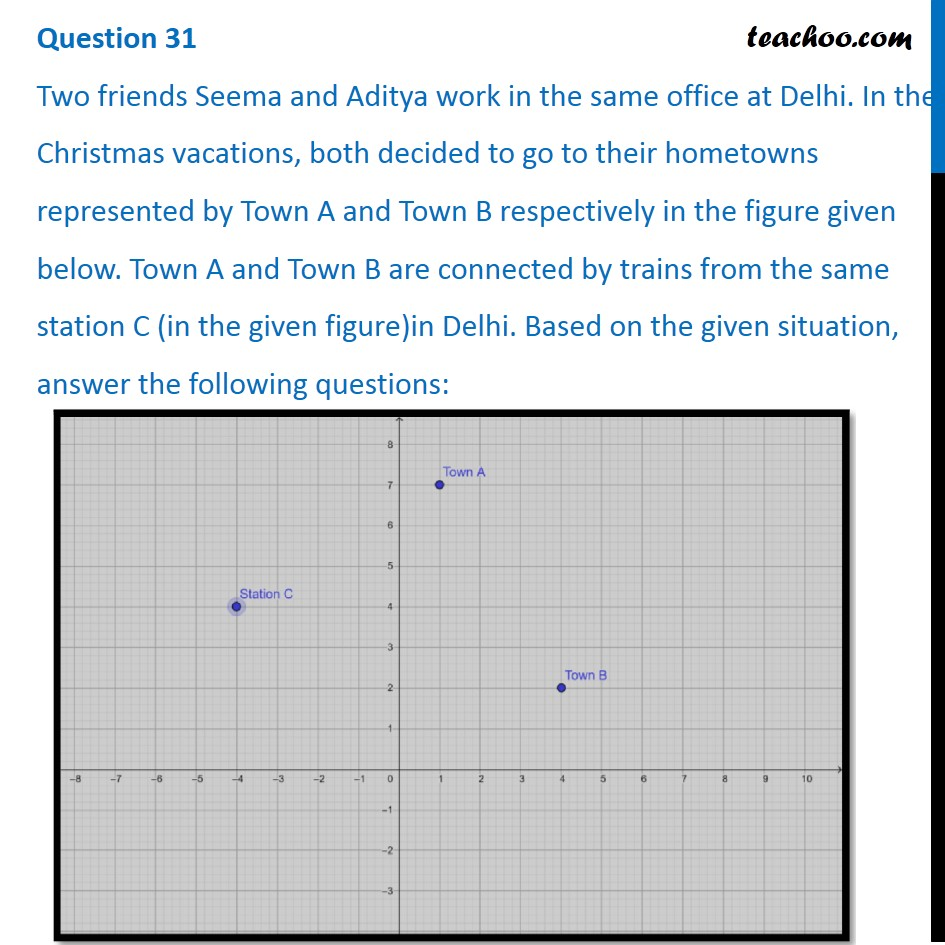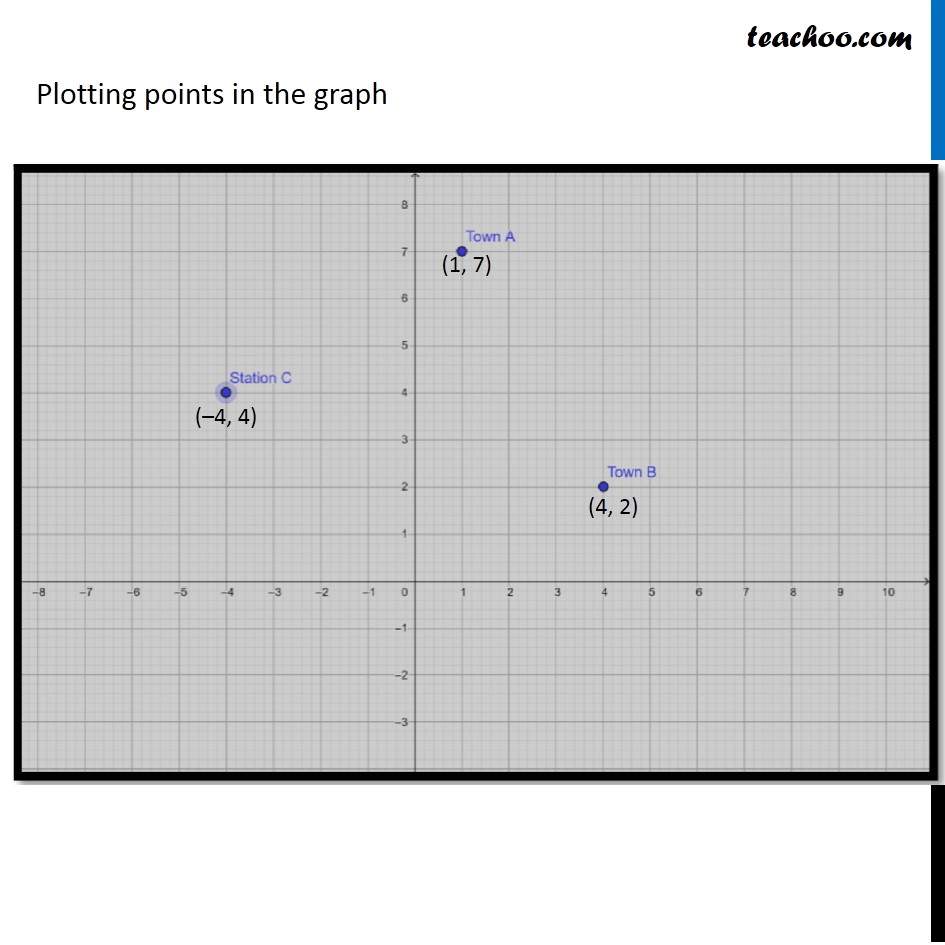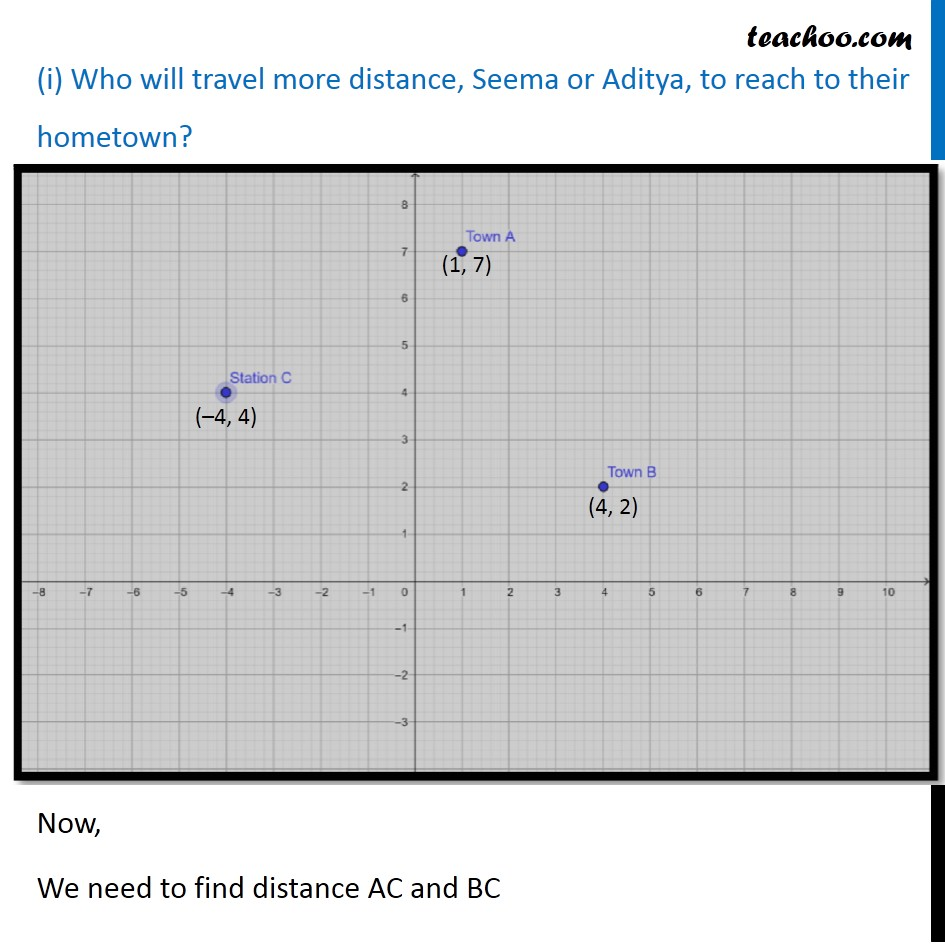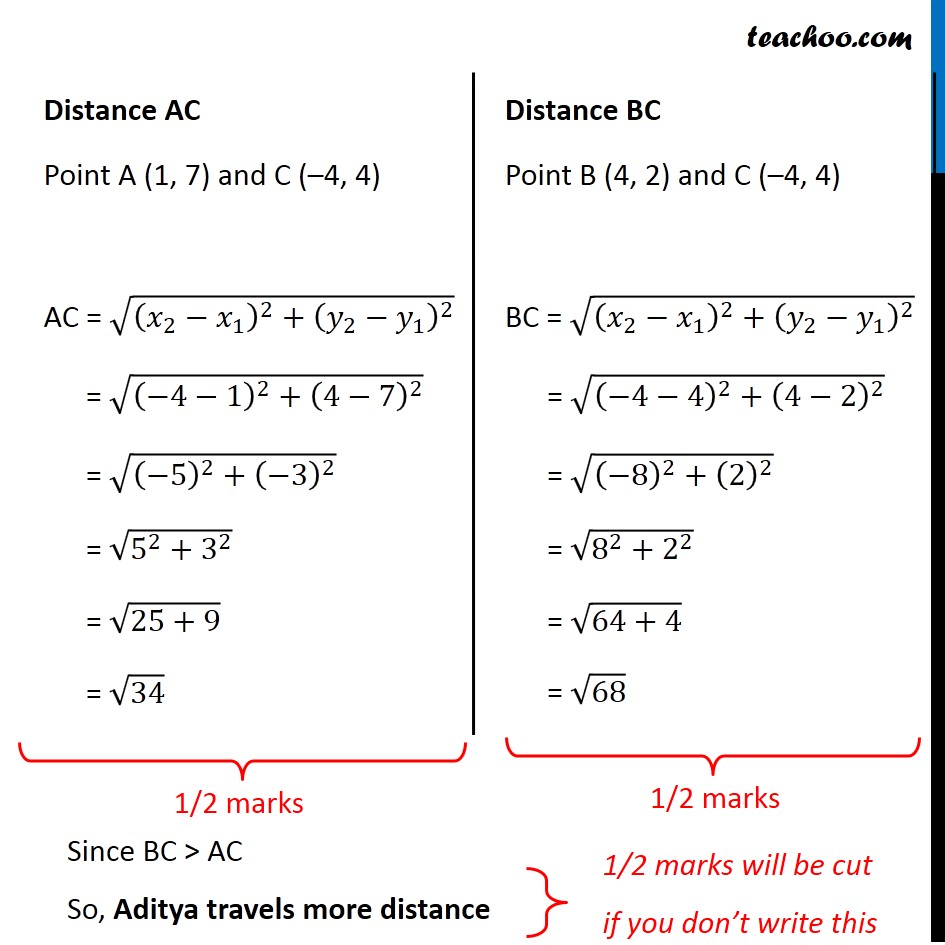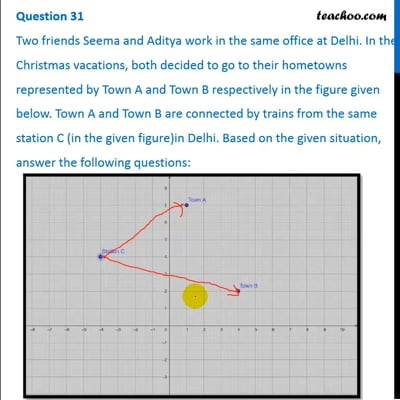This video is only available for Teachoo black users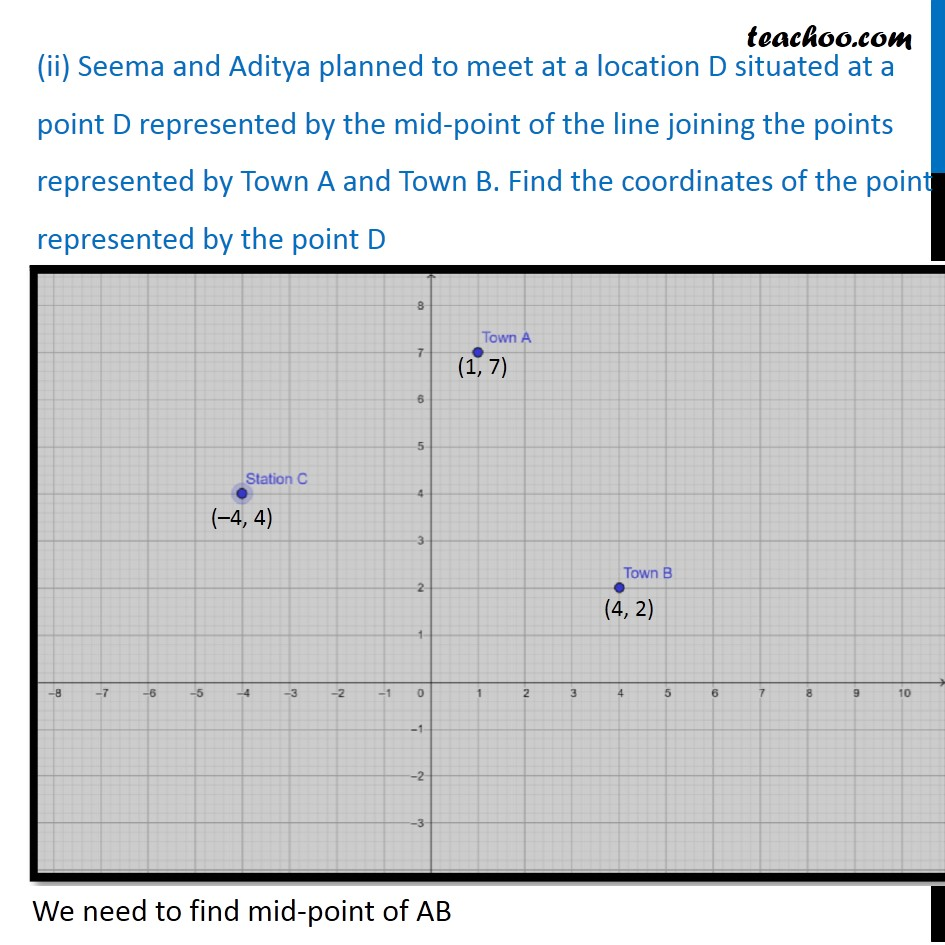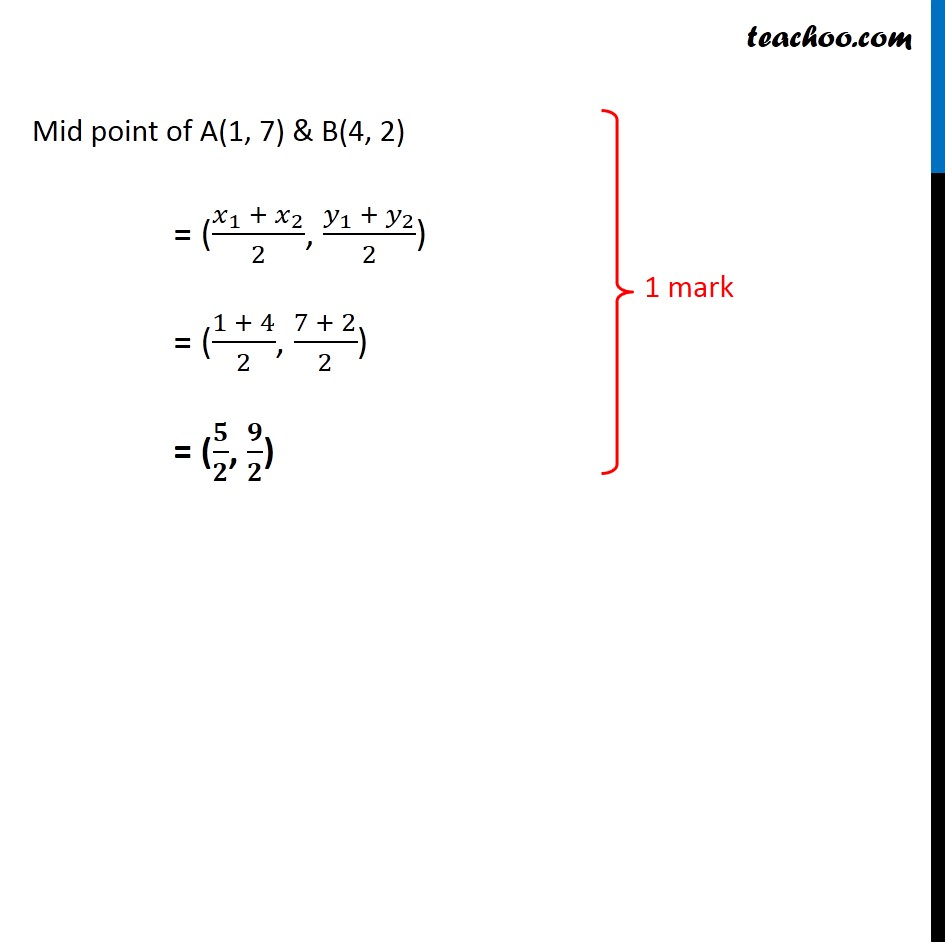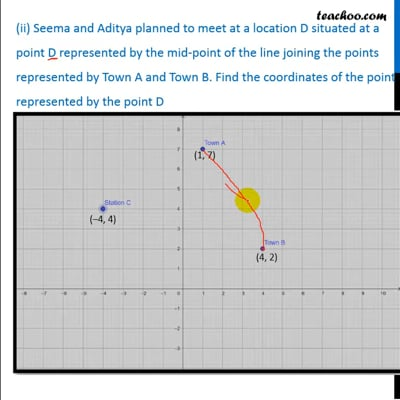This video is only available for Teachoo black users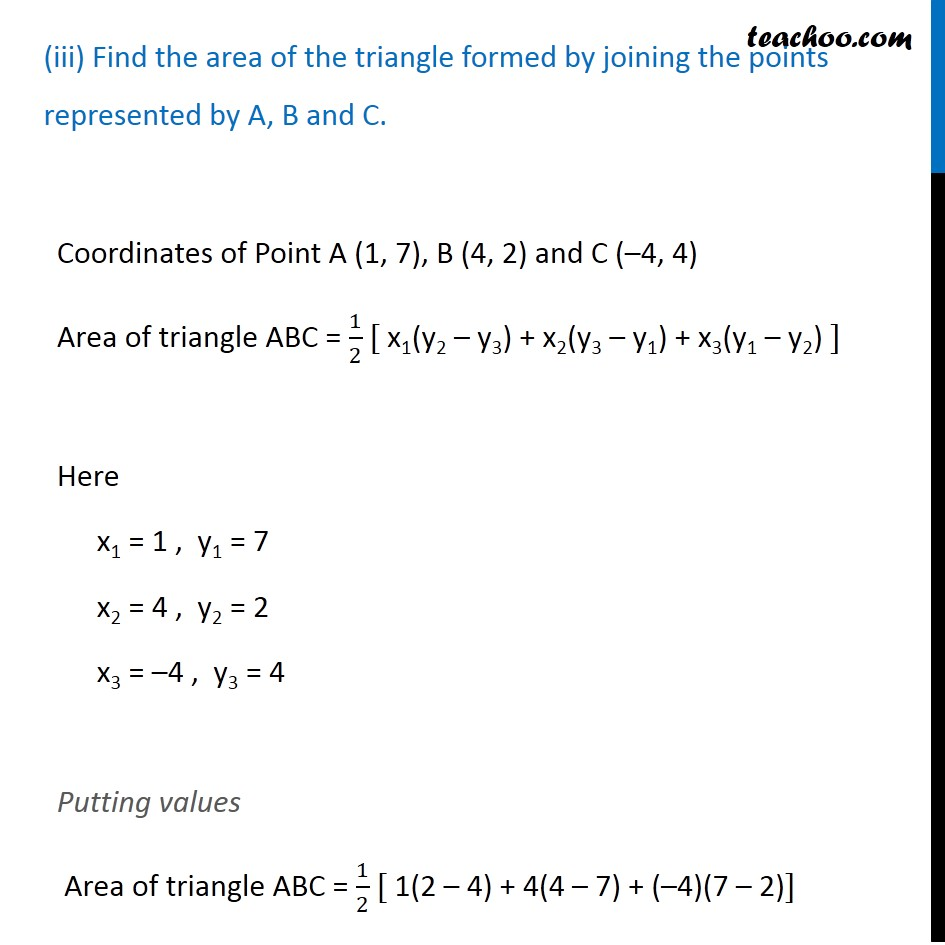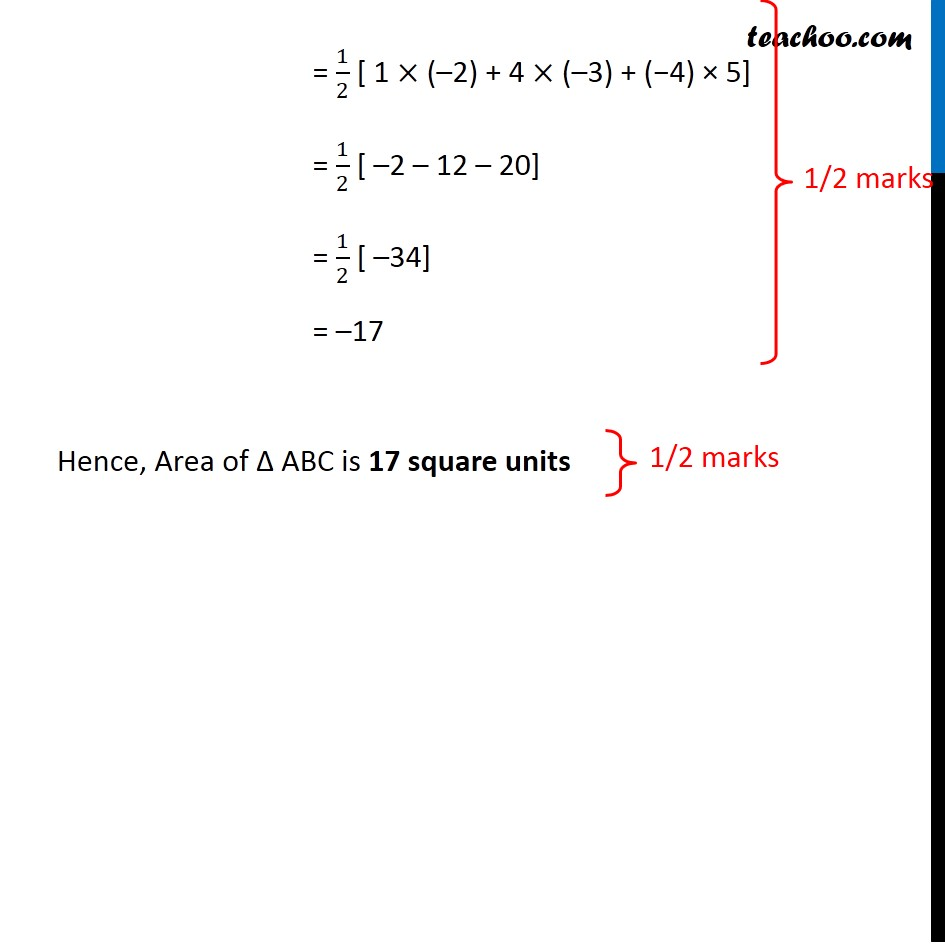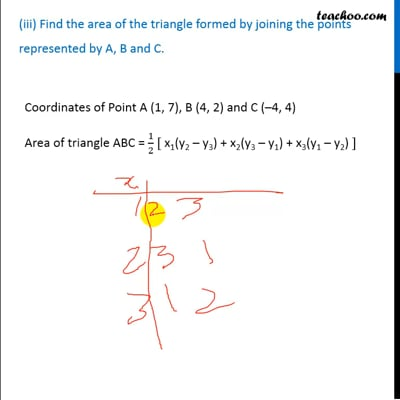This video is only available for Teachoo black users

### Transcript

Question 31 Two friends Seema and Aditya work in the same office at Delhi. In the Christmas vacations, both decided to go to their hometowns represented by Town A and Town B respectively in the figure given below. Town A and Town B are connected by trains from the same station C (in the given figure)in Delhi. Based on the given situation, answer the following questions: Plotting points in the graph (i) Who will travel more distance, Seema or Aditya, to reach to their hometown? Now, We need to find distance AC and AB Distance AC Point A (1, 7) and C (–4, 4) AC = √((𝑥_2−𝑥_1 )^2+(𝑦_2−𝑦_1 )^2 ) = √((−4−1)^2+(4−7)^2 ) = √((−5)^2+(−3)^2 ) = √(5^2+3^2 ) = √(25+9) = √34 Distance BC Point B (4, 2) and C (–4, 4) BC = √((𝑥_2−𝑥_1 )^2+(𝑦_2−𝑦_1 )^2 ) = √((−4−4)^2+(4−2)^2 ) = √((−8)^2+(2)^2 ) = √(8^2+2^2 ) = √(64+4) = √68 Since BC > AC So, Aditya travels more distance (ii) Seema and Aditya planned to meet at a location D situated at a point D represented by the mid-point of the line joining the points represented by Town A and Town B. Find the coordinates of the point represented by the point D We need to find mid-point of AB Mid point of A(1, 7) & B(4, 2) = ((𝑥_1 + 𝑥_2)/2, (𝑦_1 + 𝑦_2)/2) = ((1 + 4)/2, (7 + 2)/2) = (𝟓/𝟐, 𝟗/𝟐) (iii) Find the area of the triangle formed by joining the points represented by A, B and C. Coordinates of Point A (1, 7), B (4, 2) and C (–4, 4) Area of triangle ABC = 1/2 [ x1(y2 – y3) + x2(y3 – y1) + x3(y1 – y2) ] Here x1 = 1 , y1 = 7 x2 = 4 , y2 = 2 x3 = –4 , y3 = 4 Putting values Area of triangle ABC = 1/2 [ 1(2 – 4) + 4(4 – 7) + (–4)(7 – 2)] = 1/2 [ 1 × (–2) + 4 × (–3) + (−4) × 5] = 1/2 [ –2 – 12 – 20] = 1/2 [ –34] = –17 Hence, Area of Δ ABC is 17 square units